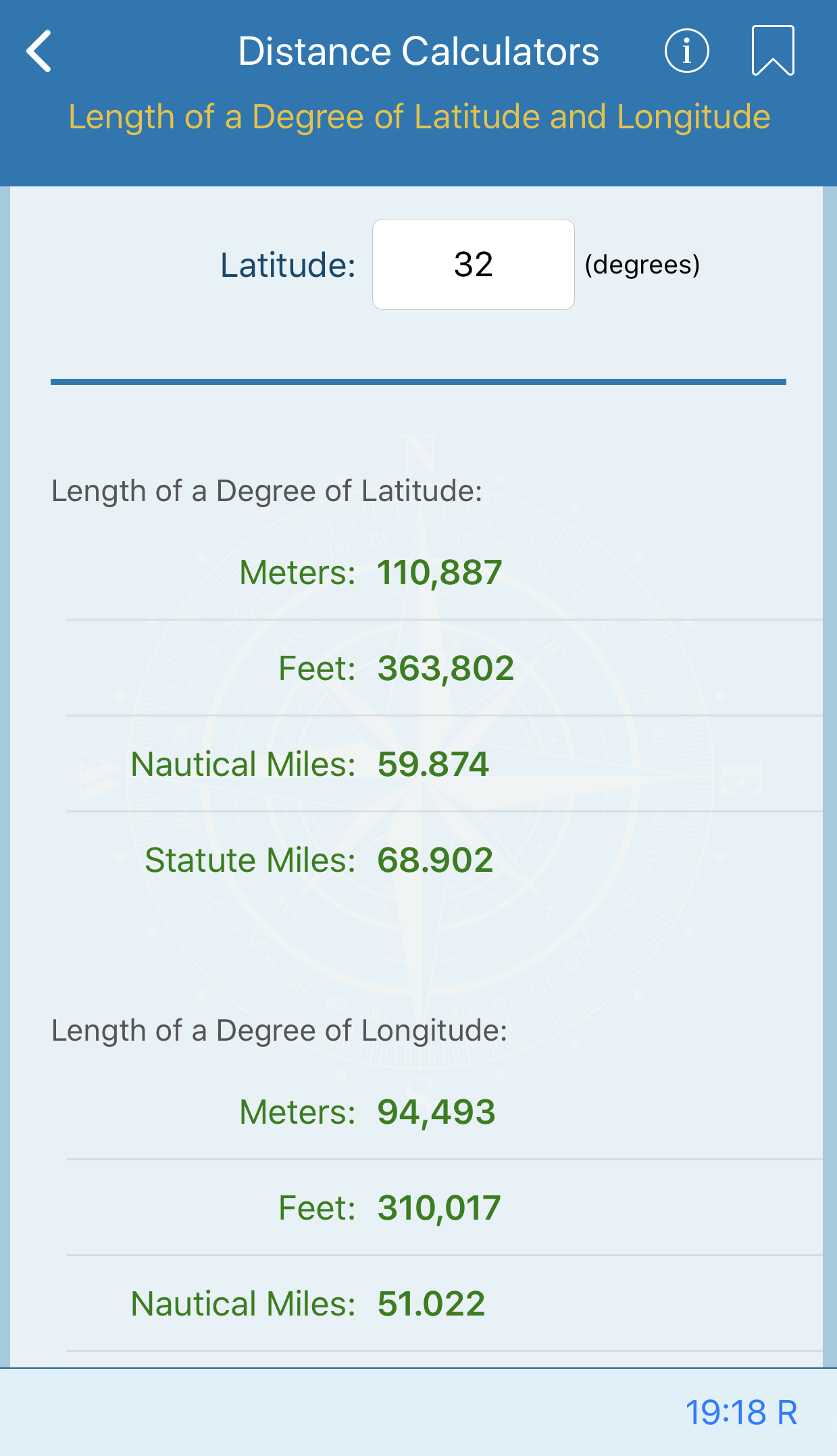free website builder

# Distance Calculators

Whether you're looking for the length of a degree, the distance of an object, horizon, dip of the sea, or meridional parts, this Nautical Calculator makes it quick and simple.## Length of a Degree of Latitude and Longitude

This calculation gives the length of one degree of latitude and longitude at intervals of 1° from the equator to the poles. Lengths are given in nautical miles, statute miles, feet, and meters.

The values were computed in meters, using the World Geodetic System ellipsoid of 1972, and converted to other units.## Speed for Measured Mile and Speed, Time, and Distance

To find the speed of a vessel on a measured nautical mile in a given number of minutes and seconds of time, enter the time in total seconds (i.e., 90 = 1 min 30 sec), and the calculation will produce speed in knots.

To find speed, time or distance, enter a value in two of the three fields, and the third will be calculated for you.## Distance of an Object by Two Bearings

To determine the distance of an object as a vessel on a steady course passes it, enter the two bearings, and the distance between them. The calculation uses plane oblique and right triangles to calculate the distance to the object at the second bearing and the distance to the object when abeam.## Distance of the Horizon

This calculation gives the distance in nautical and statute miles of the visible sea horizon for various heights of the eye in feet and meters. The actual distance varies somewhat as refraction changes. However, the error is generally less than that introduced by nonstandard atmospheric conditions. Also, the formula used contains an approximation which introduces a small error at the greatest heights tabulated.## Distance by Vertical Angle Measured Between Sea Horizon and Top of Object Beyond Sea Horizon

This calculation tabulates the distance to an object of known height above sea level when the object lies beyond the horizon. The vertical angle between the top of the object and the visible horizon is measured with a sextant and corrected for index error and dip only.## Traverse Table

This screen allows calculation of the data in the Traverse table. Enter known data in the appropriate fields and leave blank the fields you want to be calculated.

Note: At least two of the four values must be provided.## Geographic Range

This calculation gives the geographic range or the maximum distance at which the curvature of the earth permits light to be seen from a particular height of eye without regard to the luminous intensity of the light.

The geographic range depends upon the height of both the light and the eye of the observer.## Distance by Vertical Angle Measured Between Waterline at Object and Top of Object

This screen calculates the angle subtended by an object of known height lying at a particular distance within the observer’s visible horizon or vice versa.

The calculation provides the solution of a plane right triangle having its right angle at the base of the observed object and its altitude coincident with the vertical dimension of the observed object.

The solutions are based upon the following simplifying assumptions: (1) the eye of the observer is at sea level, (2) the sea surface between the observer and the object is flat, (3) atmospheric refraction is negligible, and (4) the waterline at the object is vertically below the peak of the object.## Dip of Sea Short of the Horizon

If land, another vessel, or other obstruction is between the observer and the sea horizon, use the waterline of the obstruction as the horizontal reference for altitude measurements, and substitute dip from this calculation for the dip of the horizon (height of eye correction) given in the Nautical Almanac.## Distance by Vertical Angle Measured Between Waterline at Object and Sea Horizon Beyond Object

This screen calculates the distance to an object lying within or short of the horizon when the height of eye of the observer is known. The vertical angle between the water-line at the object and the visible (sea) horizon beyond is measured and corrected for index error.

Distance in yards and feet is calculated from the corrected vertical angle and the height of eye of the observer in nautical miles.## Meridional Parts

This screen allows calculation of the meridional parts used in the construction of Mercator charts and in Mercator sailing for each minute of latitude from the equator to the poles.

Enter the latitude for which the meridional part is desired. Latitudes may either be entered as decimal degrees by only filling in the degrees field or as degrees and minutes by entering the values in the appropriate fields.